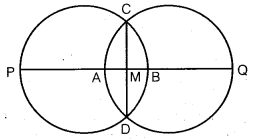# Class 6 Maths NCERT Solutions for Chapter 14 Practical Geometry Ex – 14.1

## Practical Geometry

Question 1.
Draw a circle of radius 3.2 cm.

Solution:
Steps of Construction:

1. Mark a point 0 on the paper.
2. Open the compasses for the required radius 3.2 cm.3. Keep the steel end of the compasses fixed at the point O and rotate the pencil end at 3.2 cm distance from O till it returns to the starting point P.
Then, the figure so obtained is the required circle.

Question 2.
With the same centre O, draw two circles of radii 4 cm and 2.5 cm.

Solution:
Steps of Construction:

1. Mark a point 0 on the paper.
2. Open the compasses for the radii 4 cm/2.5 cm (tumwise).3. Keep the steel end of the compasses fixed at the point 0 and rotate the pencil end at 4 cm/2.5 cm (turnwise)distance from O till it returns to their starting points respectively.
The circles obtained are the required circles.
Note: Circles with the same centre are called concentric circles.

Question 3.
Draw a circle and any two of its diameters. If you join the ends of these diameters, what is the figure obtained? What figure is obtained if the diameters are perpendicular to each other? How do you check your answer?

Solution:
Steps of Construction:

1. Draw a circle with O as centre of any radius.
2. Draw any two diameters AOB and COD.3.  Join AC, CB, BD and DA.
Clearly, the figure ACBD is a rectangle.
When the diameter AOB and COD are perpendicular to each other:Then, figure obtained on joining AC, CB, BD and DA is a square ACBD.

Question 4.
Draw any circle and mark points A, B and C such that:

(a) A is on the circle.
(b) B is in the interior of the circle.
(c) C is in the exterior of the circle.

Solution:
Steps of Construction:

1. Draw a circle with O as centre of any radius.
2. Mark point
(a) A on the circle,
(b) B in the interior of the circle, and
(c) C in the exterior of the circle.

Question 5.
Let A, B be the centres of two circles of equal radii; draw them so that each one of them passes through the centre of the other. Let them intersect at C and D.

Examine whether AB and CD are at right angles.

Solution:
Steps of Construction:

1. Mark points A and Bona paper such that AB = radii (i.e., 3 cm, say).2. With A as centre draw a circle of any radius (say, 3 cm).
3. With B as centre draw a circle of radius 3 cm as shown.
4. Let these circles intersect at C and D.
Clearly, on measuring we find ∠AMC = 90°, so AB ⊥ CD.